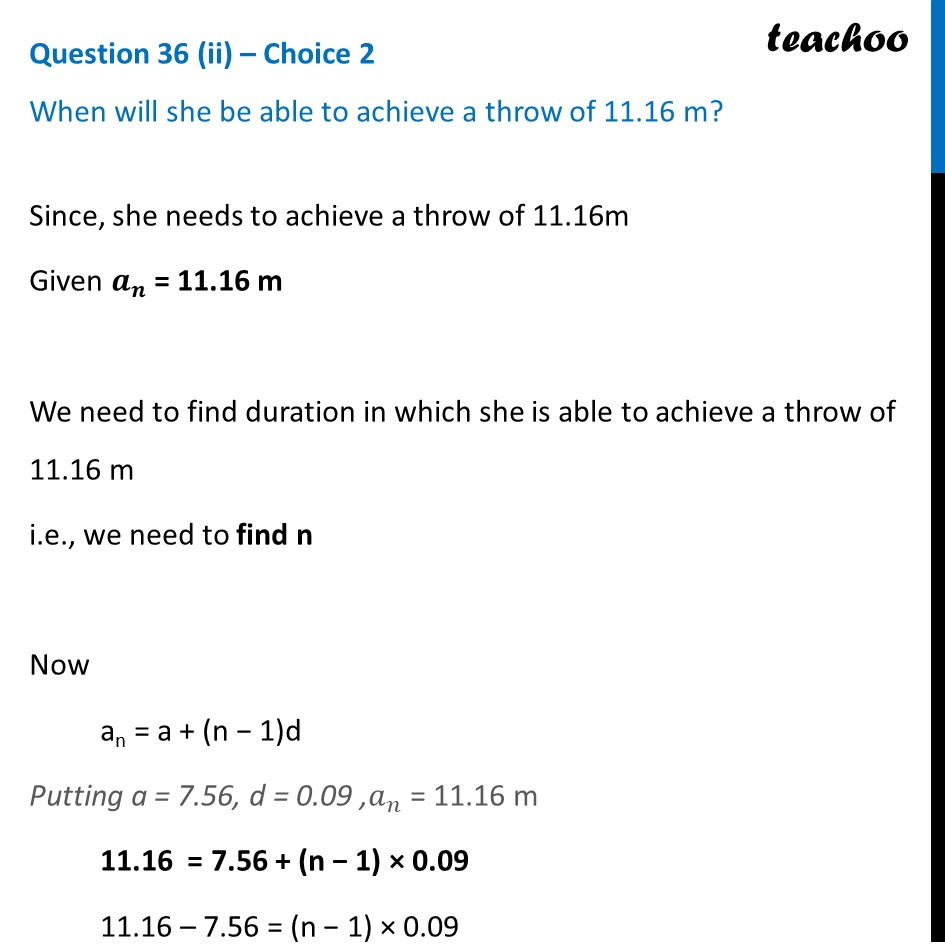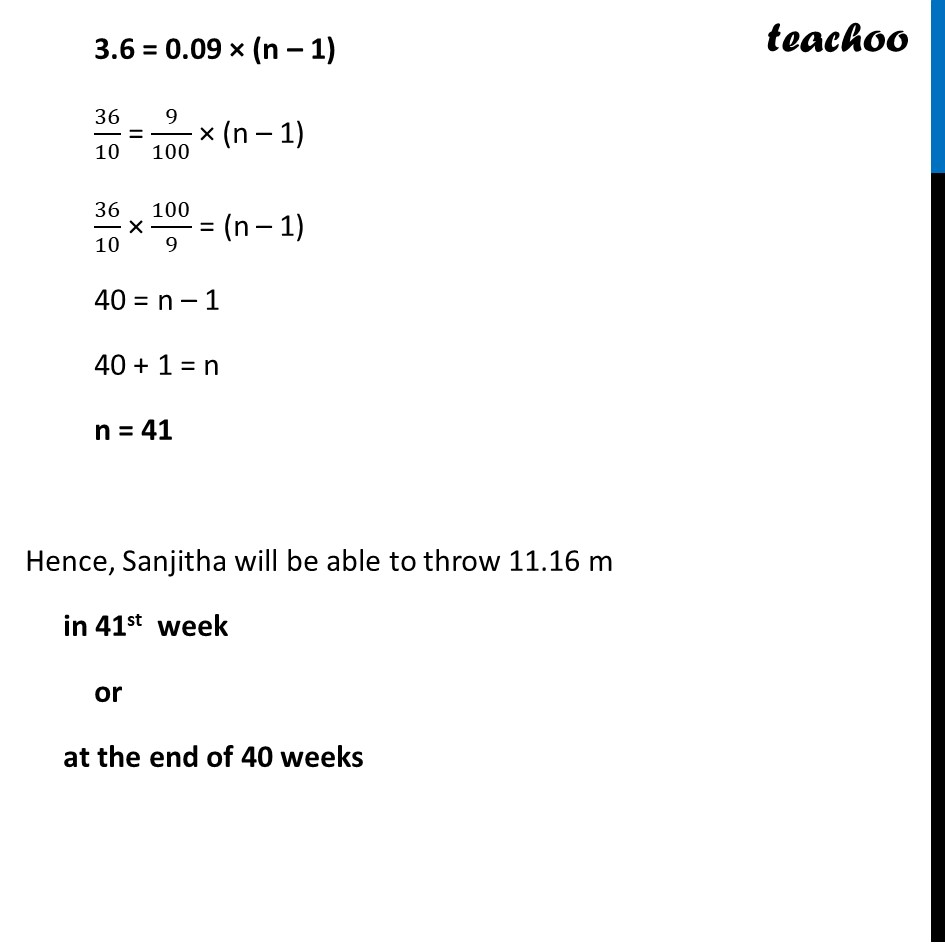CBSE Class 10 Sample Paper for 2024 Boards - Maths Standard

Class 10
Solutions of Sample Papers for Class 10 Boards

## When will she be able to achieve a throw of 11.16 m?Learn in your speed, with individual attention - Teachoo Maths 1-on-1 Class

### Transcript

Since, she needs to achieve a throw of 11.16m Given 𝒂_𝒏 = 11.16 m We need to find duration in which she is able to achieve a throw of 11.16 m i.e., we need to find n Now an = a + (n − 1)d Putting a = 7.56, d = 0.09 ,𝑎_𝑛 = 11.16 m 11.16 = 7.56 + (n − 1) × 0.09 11.16 – 7.56 = (n − 1) × 0.09 3.6 = 0.09 × (n – 1) 36/10 = 9/100 × (n – 1) 36/10 × 100/9 = (n – 1) 40 = n – 1 40 + 1 = n n = 41 Hence, Sanjitha will be able to throw 11.16 m after 42 weeks or in 41 weeks